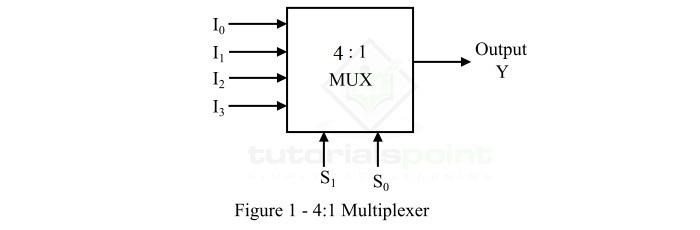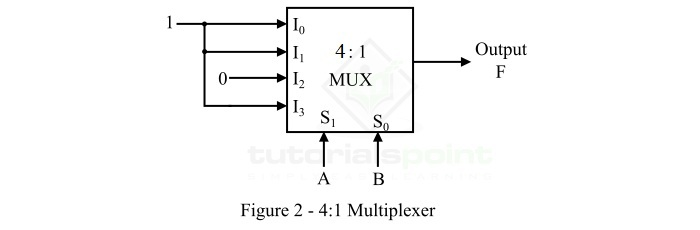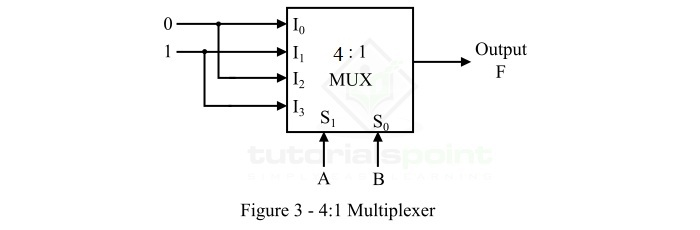# Two-Variable Function Using a 4:1 Multiplexer

Read this article to learn how you can implement a two-variable Boolean function using a 4:1 multiplexer. Let's start with a brief introduction of two-variable Boolean functions and multiplexers.

## What is a Two-Variable Boolean Function?

A two variable Boolean function is a logical expression which has two input variables. Where, each variable can take either a binary 0 or a binary 1 as its value. A two variable Boolean function can have 4 possible combinations of variables, i.e. in SOP form, $\bar{A}\bar{B},\bar{A} B,A \bar{B},AB,$ with minterm designations m0, m1, m2, and m3. In POS form,$(A+B),(A+\bar{B}),(\bar{A}+B),(\bar{A}+\bar{B})$ with maxterm designations M0, M1, M2, M3.

## What is a Multiplexer?

In digital electronics, a multiplexer, also called MUX or data selector, is a combinational logic circuit that accepts multiple data inputs and allows only one of them at a time to pass through the output line. The multiplexer has select lines to control which data input will pass through the output line. Depending upon the data input lines, there are several types of multiplexers such as 2:1 MUX, 4:1 MUX, 8:1 MUX, 16:1 MUX, and so on.

## Introduction to 4:1 Multiplexer

The block diagram of a 4:1 multiplexer is shown in Figure-1.The 4:1 multiplexer consists of 4 data input lines, i.e. I0, I1, I2, and I3, and two select lines, i.e. S0 and S1. The logic level applied to S0 and S1 determines which input data will pass through the output line.

The operation of the 4:1 multiplexer can be understood with the help of its truth table which is given below.

Select Lines Output
S1 S0 Y
00I0
01I1
10I2
11I3

As we know, a two variable Boolean function has 4 possible combinations of input variables. Therefore, we can realize any two variable Boolean function using a 4:1 multiplexer.

Now, let us discuss the implementation of a two variable Boolean function using 4:1 MUX along with solved examples.

## Implementation of a Two-Variable Function using a 4:1 Multiplexer

The implementation of a two variable Boolean function using a 4:1 multiplexer involves the following steps −

• Step 1 − Draw the truth table for the given two variable Boolean function.

• Step 2 − The two input variables A and B are applied to the select lines S1 and S0 respectively.

• Step 3 − Connect logic 1 to those data input lines where the function is 1 in the truth table.

• Step 4 − Connect logic 0 to all the remaining data input lines.

Now, let us understand the realization of a two variable Boolean function using a 4:1 multiplexer with the help of an example.

## Example 1

Use a 4:1 multiplexer to implement the following two variable logic function.

$$F(A+B)=\sum m(0, 1, 3)$$

### Solution

The truth table of the 4:1 multiplexer for the given logic function is as follows −

Select Lines Output
S1 = A S0 = B Y
001
011
100
111

Using this truth table, we can draw the logic block diagram to realize the function F using a 4:1 MUX which is shown in Figure-2.### Explanation

Here, the inputs A and B are applied to the select lines S1, and S0 respectively. From the truth table, it is clear that the function F = 1, when AB = 00, 01, 11. Thus, we connect logic 1 to the data input lines I0, I1, and I3, and the logic 0 is connected to the data input line I2.

## Example 2

Implement the following two variable logic function by using a 4:1 MUX.

$$F(A,B)=\sum m(1, 3)$$

### Solution

The truth table of the 4:1 multiplexer for the given logic function is as follows,

Select Lines Output
S1 = A S0 = B Y
000
011
100
111

Using this truth table, we can draw the logic block diagram to realize the function F using a 4:1 MUX which is shown in Figure-3.### Explanation

Here, the inputs A and B are applied to the select lines S1, and S0 respectively. From the truth table, it is clear that the given Boolean function F = 1, when AB = 01, 11. Hence, we connect logic 1 to the data input lines I1 and I3, and the logic 0 is connected to the remaining data input lines, i.e. I0 and I2.

## Conclusion

In this way, we can implement a given two variable logic function with the help of a 4:1 multiplexer. Try to solve the following tutorial problems on implementation of a two variable Boolean function by using a 4:1 multiplexer to understand the concept in more depth.

Q. 1 − Use a 4:1 multiplexer to implement the following two variable Boolean function.

$$F(x,y)=\sum m(0, 1)$$

Q. 2 − Implement the following two variable Boolean function using a 4:1 multiplexer.

$$F(A,B)=\sum m(1,2,3)$$

Q. 3 − Implement the following Boolean function by using 4:1 MUX.

$$F(A,B)=\sum m(0)$$

Updated on: 19-Apr-2023

659 Views